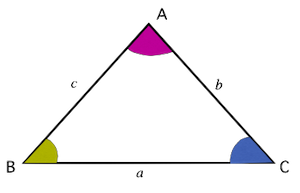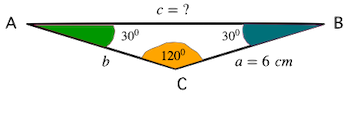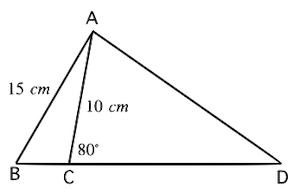407 493 6601

# Precalculus - The Law of Sines

## Introduction

• A triangle in which none of its interior angles are $90°$ is called a non-right triangle or oblique triangle.
• Since trigonometric ratios can only be applied to right triangles, how can we solve non-right triangles? [Note that solving a triangle means finding the measurement of its angles and sides].
• Here comes the role of laws of sines. It relates the sides and angles of non-right triangles.

## The Sine Rule

• Consider a triangle ABC, as shown in the figure below.• The Law of Sines or Sine rule states that:

$\frac{a}{\mathrm{sin}A}=\frac{b}{\mathrm{sin}B}=\frac{c}{\mathrm{sin}C}$,  or  $\frac{\mathrm{sin}A}{a}=\frac{\mathrm{sin}B}{b}=\frac{\mathrm{sin}C}{c}$, where

$a=$the side opposite to angle A

$b=$the side opposite to angle B

$c=$the side opposite to angle C

• The Law of Sines can be applied in the following two cases:

(a) when two angles and one side (AAS/ASA) are given, or

(b) when two sides and a non-included angle (SSA) are given.

• The situations in which either all the sides of a triangle (SSS) are given or any two sides and included angle between them are given (SAS), the Law of Sines can't be applied.

## Solved Examples

Example 1: In $∆ABC$, $\angle B=30°$$\angle C=120°$, and side . Find the measure of side $AB$.

Solution: In this problem, two angles and a side is given (ASA). We can find the third angle also. Let us first draw a triangle with the given data.Applying the Sine rule in the given triangle:

$\frac{a}{\mathrm{sin}A}=\frac{b}{\mathrm{sin}B}=\frac{c}{\mathrm{sin}C}$

or, $\frac{6}{\mathrm{sin}30°}=\frac{b}{\mathrm{sin}30°}=\frac{c}{\mathrm{sin}120°}$

or, $12=2b=\frac{2c}{\sqrt{3}}$  $=6\sqrt{3}$

Therefore, the measure of side AB is .

Example 2: In the figure shown below, $m\angle ACD=80°$ and . Find the measure of angle B.Solution:

$m\angle ACB=180°-80°=100°$

## Cheat Sheet

For any triangle ABC (acute, obtuse, or right), if $a$$b$ and $c$ are the lengths of three sides opposite to $\angle A$, $\angle B$ and $\angle C$, respectively, then the sides and angles of the triangle are related as mentioned below:

• The law of Sines or Sine rule:

$\frac{a}{\mathrm{sin}A}=\frac{b}{\mathrm{sin}B}=\frac{c}{\mathrm{sin}C}$  or  $\frac{\mathrm{sin}A}{a}=\frac{\mathrm{sin}B}{b}=\frac{\mathrm{sin}C}{c}$

## Blunder Areas

• The Laws of Sines can be used in any triangle (not just right-angled triangles) except when there is ambiguity.
• The sine rule can't be applied in cases in which two sides and the included angle between them (SAS) are given, or all three sides of the triangle (SSS) are given. In such cases, we must use the Law of Cosines.